+关注继续查看

# 一、前言

class Date
{
private:
int _year;
int _month;
int _day;

public:
void Init(int year, int month, int day)
{
_year = year;
_month = month;
_day = day;
}

void Print()
{
cout << _year << '-' << _month << '-' << _day << endl;
}
};


# 二、类的六个默认成员函数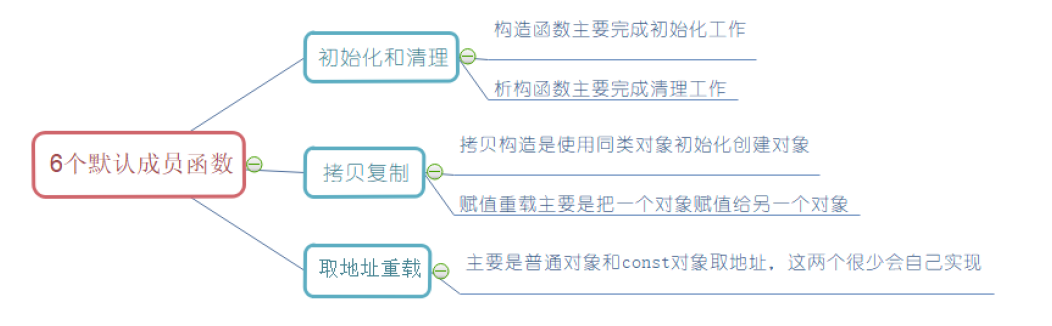# 三、构造函数

class Date
{
private:
int _year;
int _month;
int _day;

public:
void Init(int year, int month, int day)
{
_year = year;
_month = month;
_day = day;
}

void Print()
{
cout << _year << '-' << _month << '-' << _day << endl;
}
};


## 1、概念

? ?构造函数是一个特殊的成员函数，名字与类名相同，创建类类型对象时由编译器 自动调用，以保证每个数据成员都有 一个合适的初始值，并且在对象整个生命周期内只调用一次。

class Date
{
public:
Date()
{
_year = 0;
_month = 1;
_day = 1;
}

Date(int year, int month, int day)
{
_year = year;
_month = month;
_day = day;
}

private:
int _year;
int _month;
int _day;
};

int main()
{
Date d1; // 自动调用第一个构造函数
Date d2(2023, 1, 31); // 传参调用第二个构造函数

return 0;
}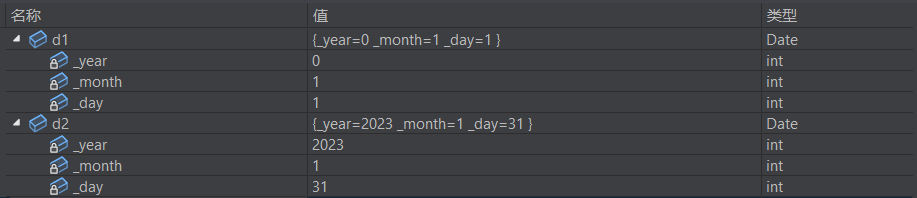Date(int year = 0, int month = 1, int day = 1)
{
_year = year;
_month = month;
_day = day;
}
// 调用
Date d1;
Date d2(2023, 1);
Date d3(2023, 1, 31);

## 2、特性

? ? ? ?函数名与类名相同。

? ? ? ?无返回值。

? ? ? ?对象 实例化 时编译器 自动调用 对应的构造函数。

? ? ? ?构造函数可以重载。

? ?5）如果类中没有显式定义构造函数，则C++编译器会自动生成一个无参的默认构造函数；一旦用户显式定义，编译器将不再生成默认无参构造函数

int/char/double/内置类型数组），自定义类型（struct/class定义的类型）。

? ?下面我们验证：若用户定义了构造函数，则编译器则不生成默认无参构造函数，若调用方式错误，则会报错。

class A
{
public:
A()
{
cout << "A()" << endl;
_a = 0;
}
private:
int _a;
};

class Date
{
public:
Date(int year = 0, int month = 1, int day = 1)
{
_year = year;
_month = month;
_day = day;
}

private:
int _year;
int _month;
int _day;

A _aa;
};

int main()
{
Date d1;

return 0;
}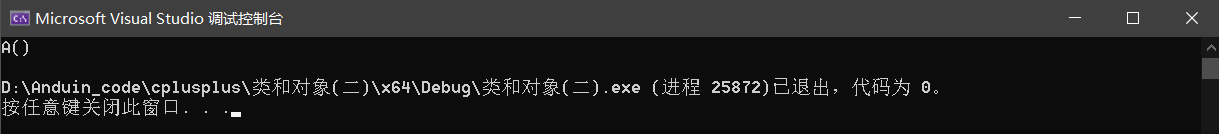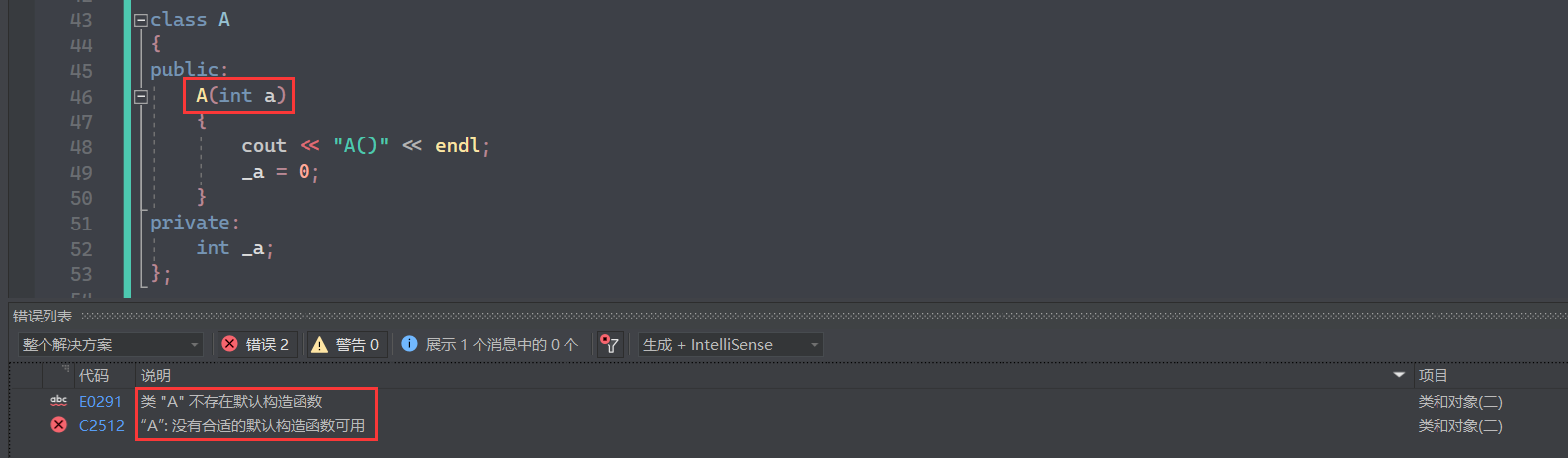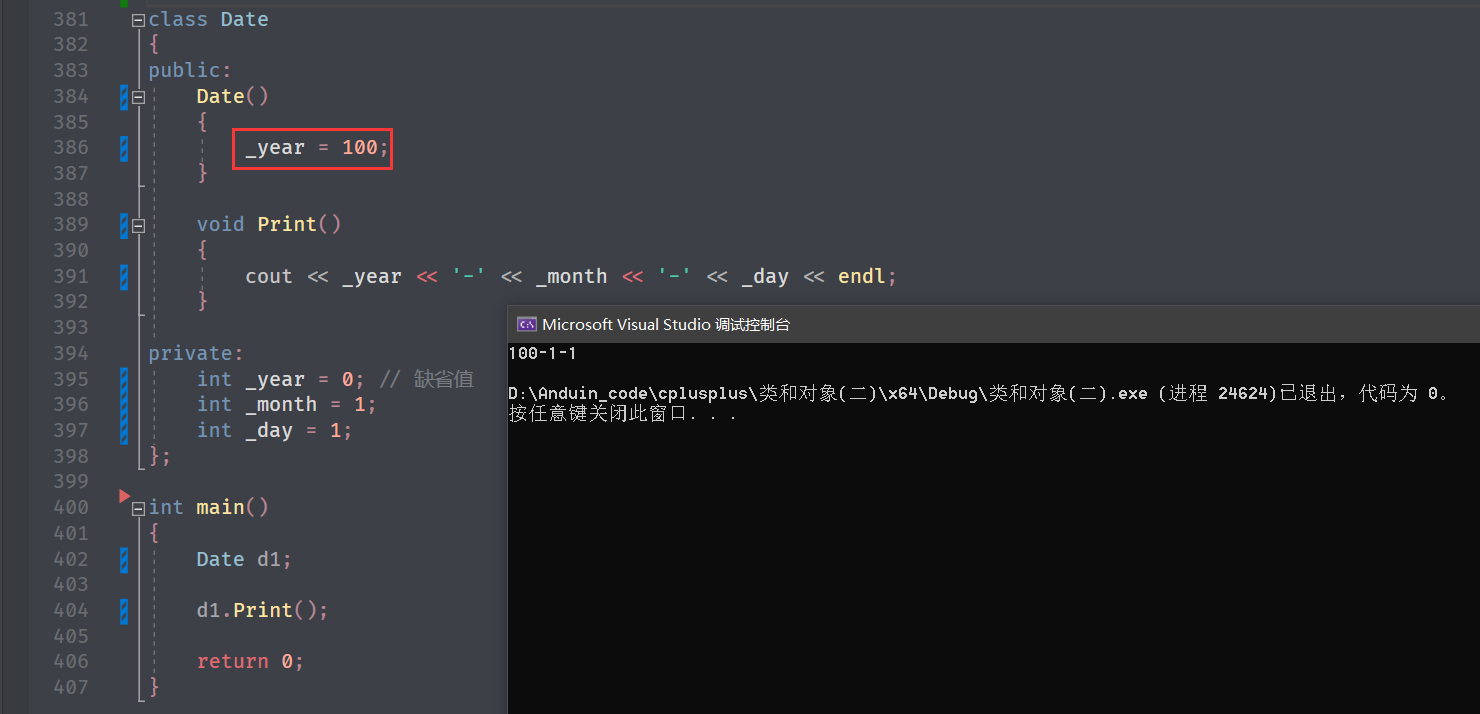_year 给了 100 ，所以有值，其他的没有给，所以用默认值（缺省值）。

6）无参的构造函数和全缺省的构造函数都称为默认构造函数，并且默认构造函数只能有一个（无参构造函数、全缺省构造函数、没写编译器默认生成的构造函数，都可以认为是默认构造函数）

# 四、析构函数

## 2、特性

~Date()
{
// Date 类没有资源需要清理，所以当前 Date 类不写闲析构函数都可以
cout << "~Date()" << endl;
}

class Stack
{
public:
Stack(int capacity = 4) // 构造函数，缺省1参数，不传参默认给 4
{
_a = (int*)malloc(sizeof(int) * capacity);
if (_a = nullptr)
{
cout << "malloc fail" << endl;
exit(-1);
}

_top = 0;
_capacity = capacity;
}

private:
int* _a;
size_t _top;
size_t _capacity;
};

int main()
{
Stack s1;
Stack s2;

return 0;
}


~Stack()
{
free(_a);
_a = nullptr;
_top = _capacity = 0;
}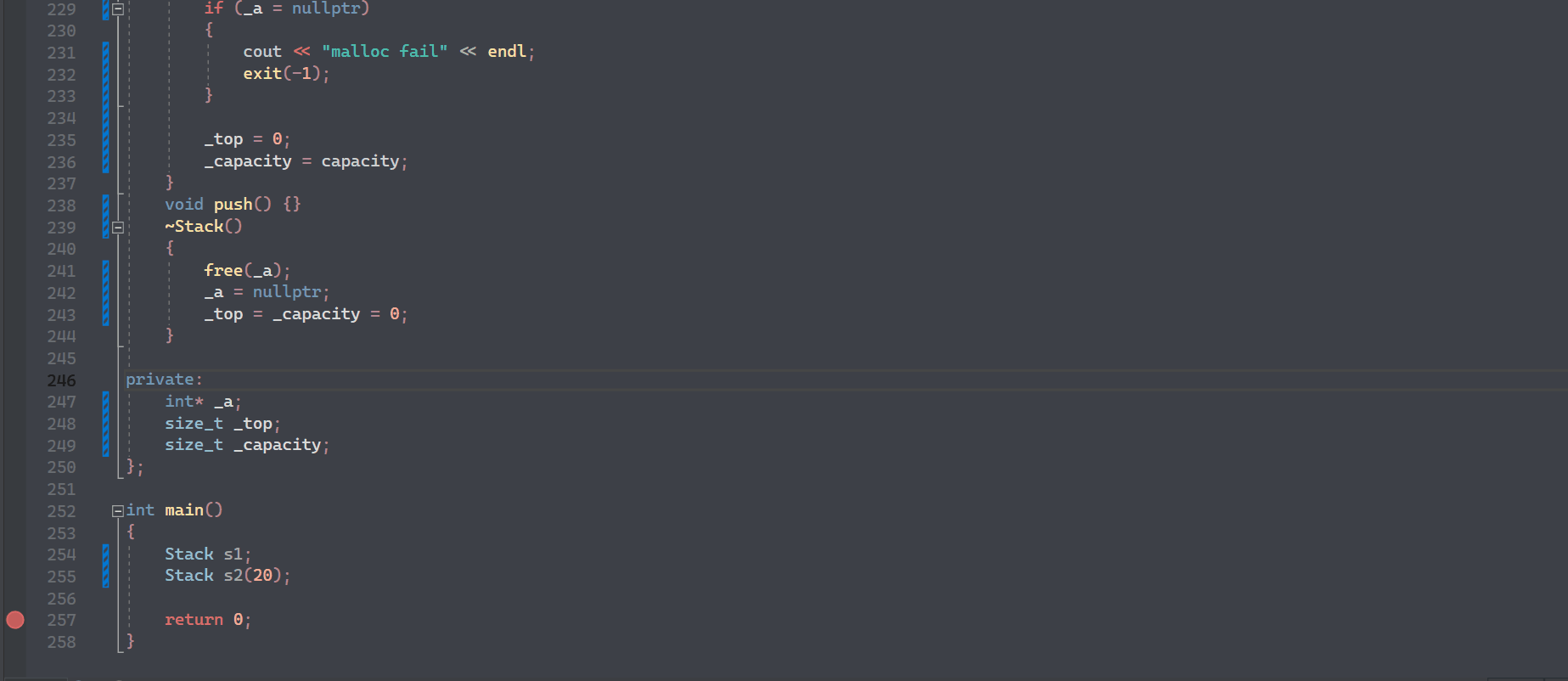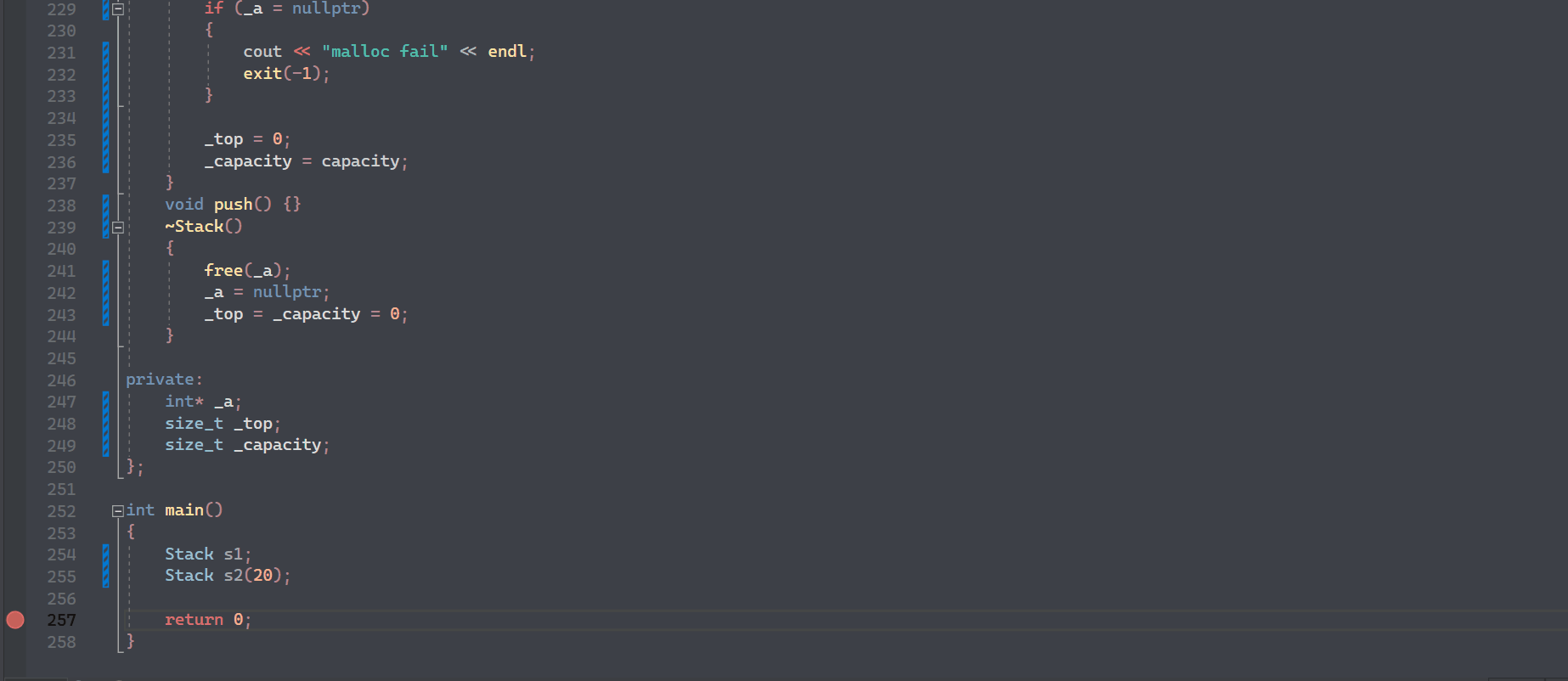? ?可能有小伙伴会疑惑，C++ 为什么多此一举搞自动生成析构函数，它又不对内置类型处理，没什么用啊？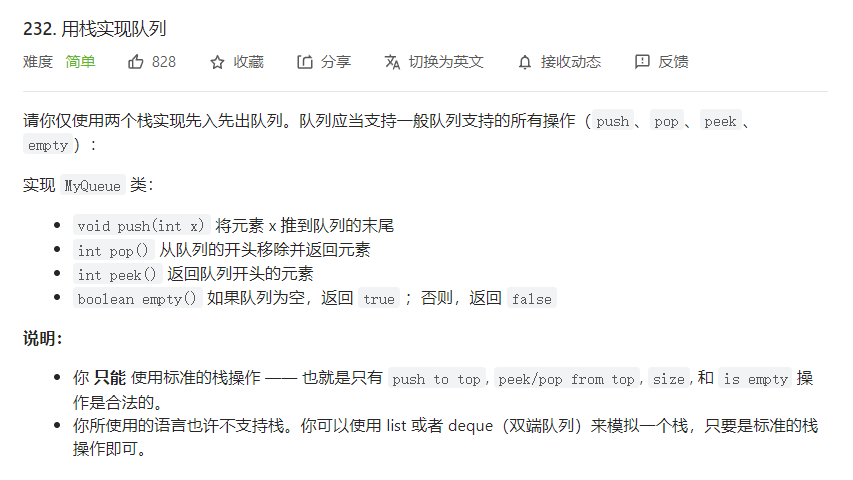class Stack
{
public:
Stack(int capacity = 4) // 构造
{
_a = (int*)malloc(sizeof(int) * capacity);
if (_a = nullptr)
{
cout << "malloc fail" << endl;
exit(-1);
}
_top = 0;
_capacity = capacity;
}
~Stack() // 析构
{
free(_a);
_a = nullptr;
_top = _capacity = 0;
}
private:
int* _a;
size_t _top;
size_t _capacity;
};

class MyQueue
{
public:
void push(int x) {}
private:
Stack pushST;
Stack popST;
};

int main()
{
MyQueue mq; // 自动调用构造和析构
return 0;
}


? ? ? ?析构函数名是在类名前加上字符 ~

? ? ? ?无参数无返回值类型

? ? ? ?一个类只能有一个析构函数（无参不构成重载）; 若未显式定义，系统会自动生成默认的析构函数

? ? ? ?对象生命周期结束时（局部变量出作用域），C++编译系统自动调用析构函数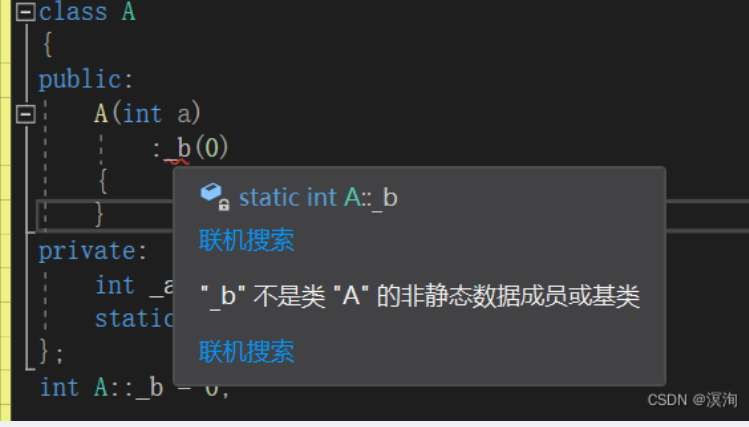C++ 类和对象（静态的static、友元、内部类、匿名对象、explicit）知识点+完整思维导图+实操图+深入细节通俗易懂建议收藏（二）
C++ 类和对象（静态的static、友元、内部类、匿名对象、explicit）知识点+完整思维导图+实操图+深入细节通俗易懂建议收藏（二）
17 0C++ 类和对象（静态的static、友元、内部类、匿名对象、explicit）知识点+完整思维导图+实操图+深入细节通俗易懂建议收藏（一）
C++ 类和对象（静态的static、友元、内部类、匿名对象、explicit）知识点+完整思维导图+实操图+深入细节通俗易懂建议收藏（一）
11 0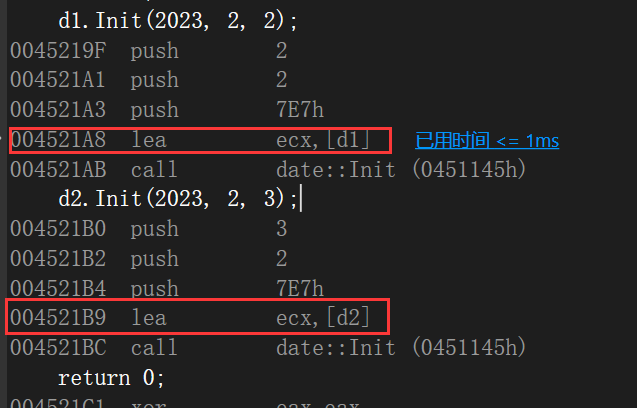C++类和对象（一）
c++类和对象 (一) 1. 面向对象和面向过程初步认识 C语言是面向过程的，关注的是过程，分析出求解问题的步骤，通过函数调用逐步解决问题。比如洗衣服这个场景：先拿盆子，再防水到盆子中，然后放衣服和洗衣粉，手搓，换水，再手搓，直到水清为止，拧干， 晒衣服。C++语言是面向对象的，关注的是对象，将一件事情拆分成不同的对象，靠对象之间的交互完成。总共有四个对象：衣服，人，洗衣机，洗衣粉
14 0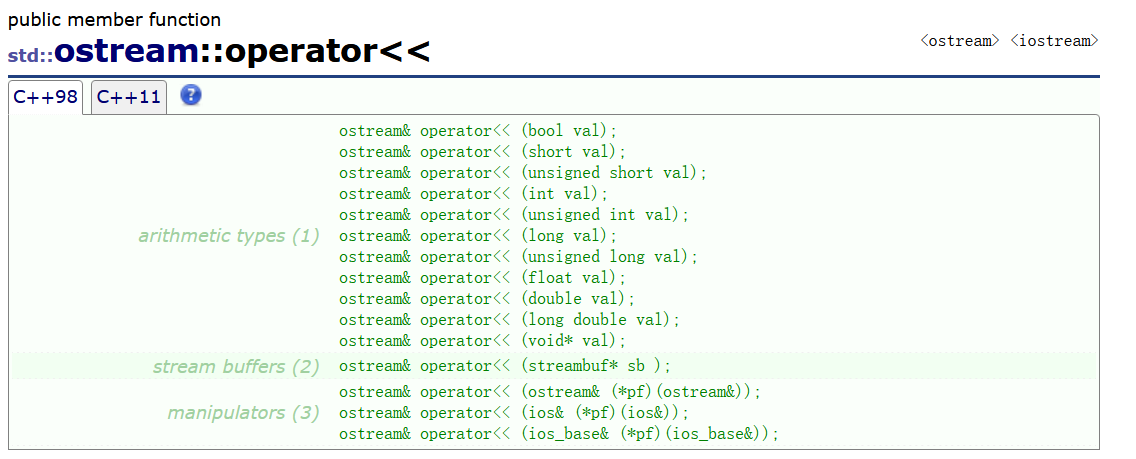C++类和对象（二）(2)
C++类和对象（二） 1.六个默认成员函数 如果一个类中什么成员都没有，简称为空类。空类中真的什么都没有吗？并不是，任何类在什么都不写时，编译器会自动生成以下6个默认成员函数。默认成员函数：用户没有显式实现，编译器会生成的成员函数称为默认成员函数。 六个默认成员函数：构造函数（初始化工作）、析构函数（清理工作）、拷贝构造（使用同类对象初始化创建对象）、赋值重载（把一个对象赋值给另外一个对象）、取地址重载（普通对象和const对象取地址）
11 0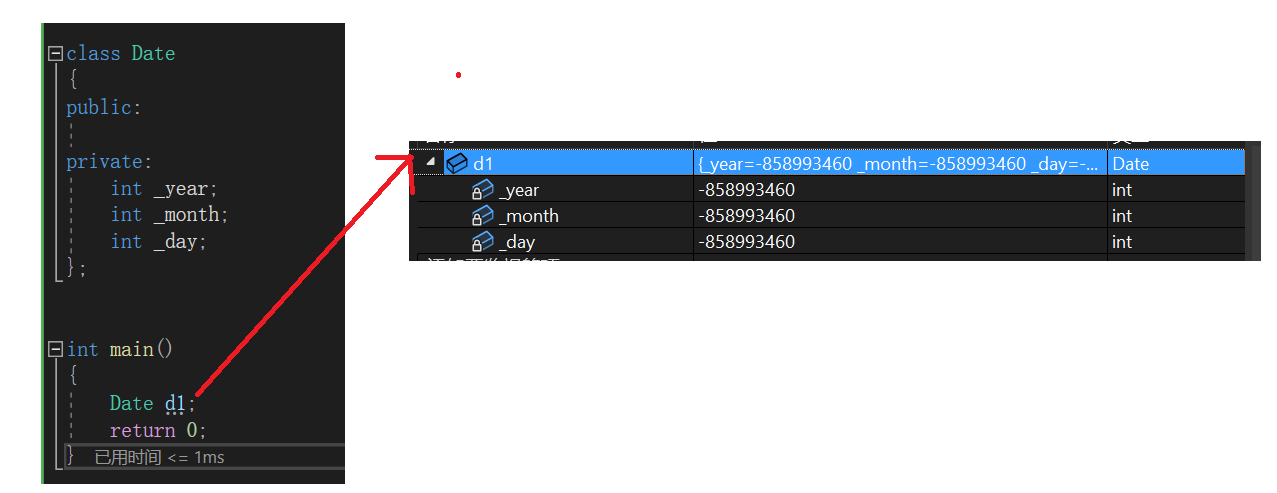C++类和对象（二）（1）
C++类和对象（二） 1.六个默认成员函数 如果一个类中什么成员都没有，简称为空类。空类中真的什么都没有吗？并不是，任何类在什么都不写时，编译器会自动生成以下6个默认成员函数。默认成员函数：用户没有显式实现，编译器会生成的成员函数称为默认成员函数。 六个默认成员函数：构造函数（初始化工作）、析构函数（清理工作）、拷贝构造（使用同类对象初始化创建对象）、赋值重载（把一个对象赋值给另外一个对象）、取地址重载（普通对象和const对象取地址）
10 0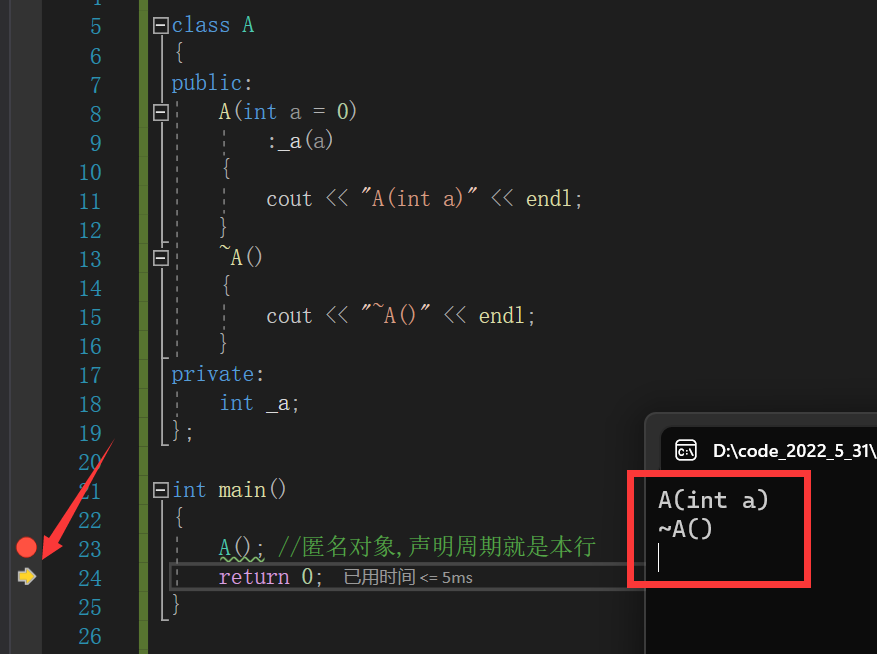C++类和对象（三）
C+类和对象（三） 1. 再聊构造函数 1.1 构造函数体内赋值
9 0c++类和对象重要巩固练习-------日期类对象
c++类和对象重要巩固练习-------日期类对象
18 0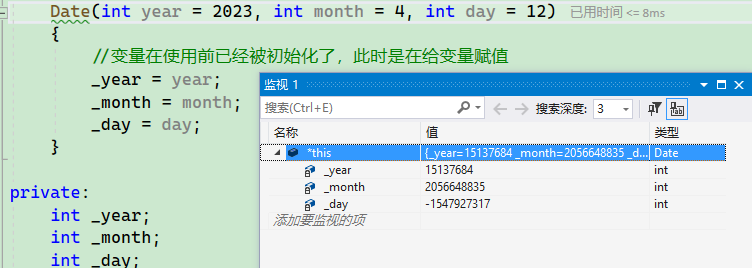C++类和对象（下）
C++类和对象（下）
22 0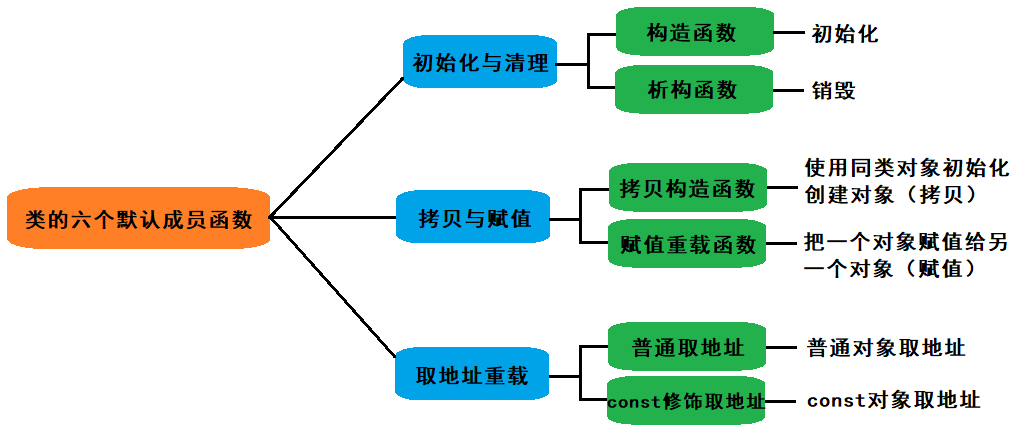C++类和对象（中）
C++类和对象（中）
22 0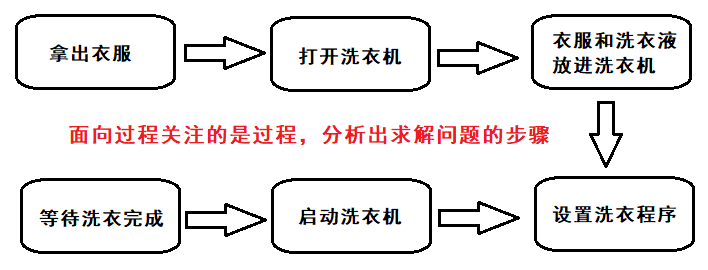C++类和对象（上）
C++类和对象（上）
18 0

Java面向对象编程10864148

GPON Class C++ SFP OLT Transce